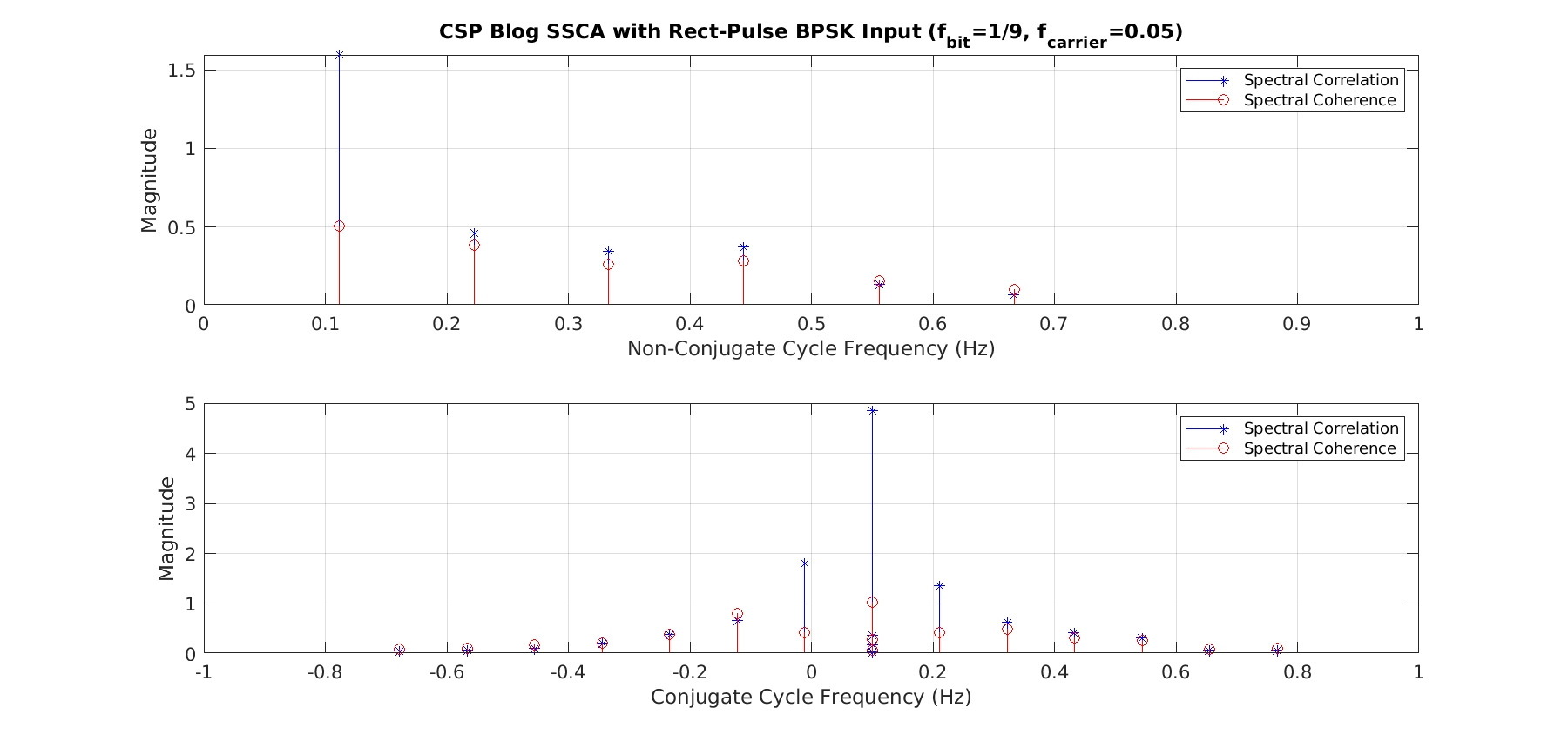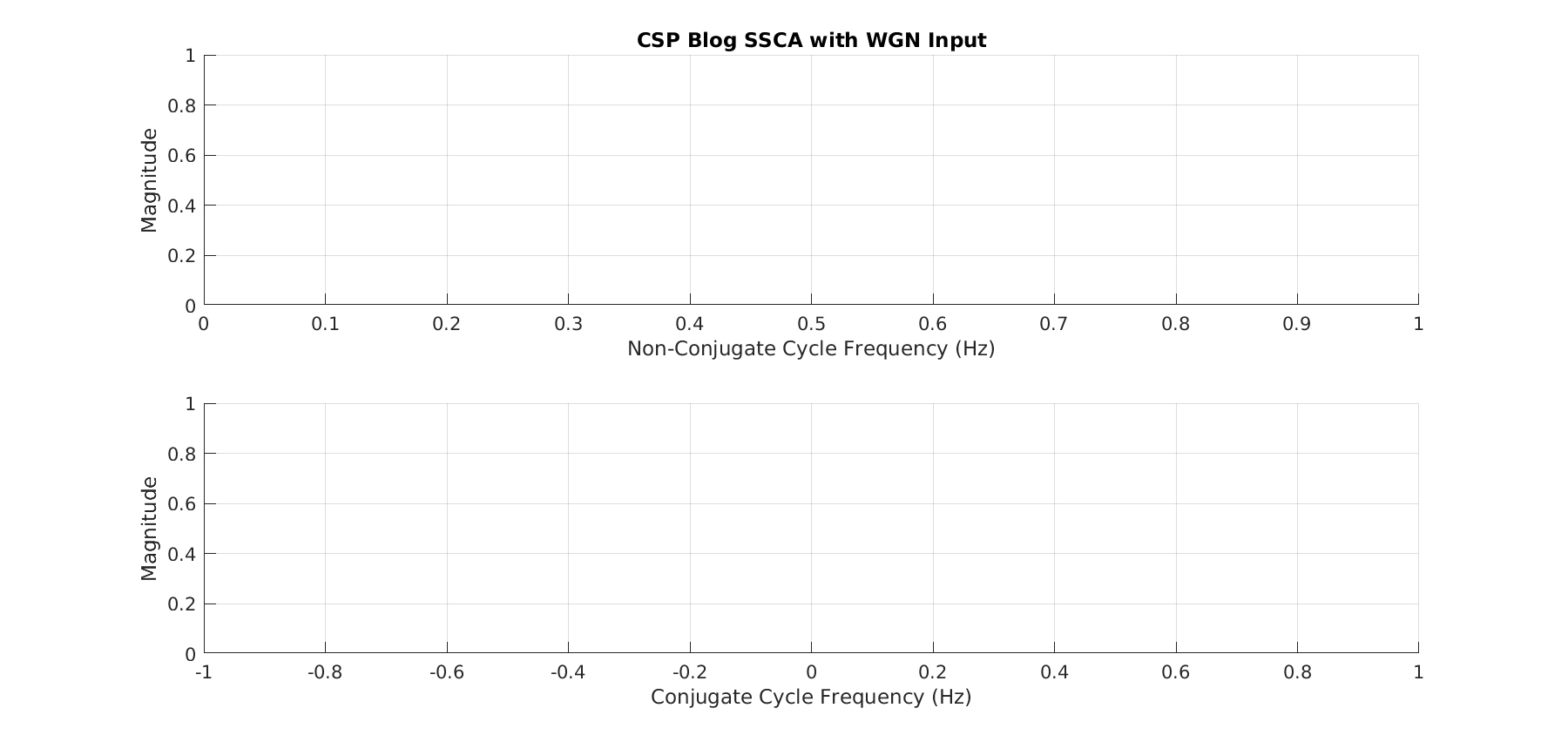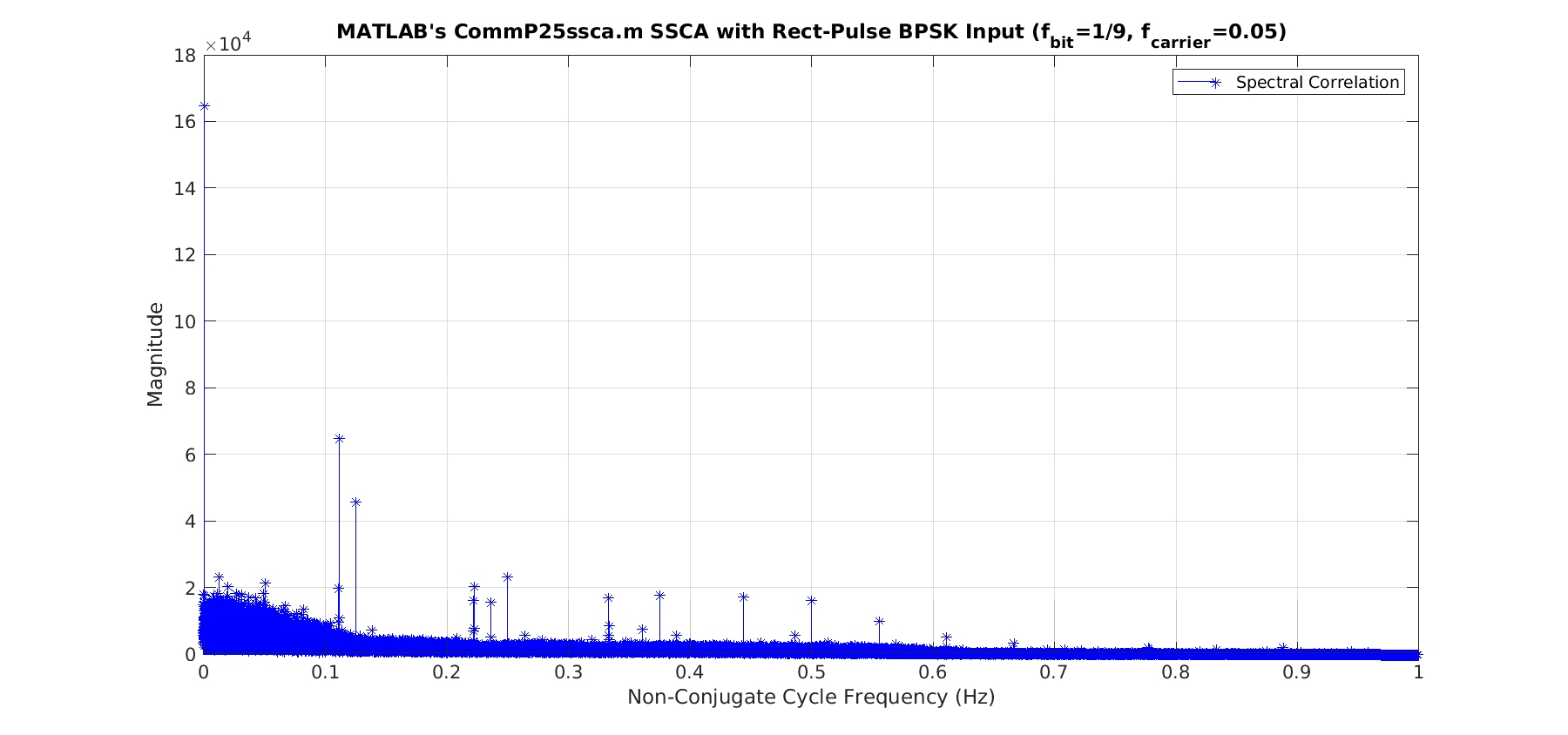# MATLAB’s SSCA: commP25ssca.m

In this short post, I describe some errors that are produced by MATLAB’s strip spectral correlation analyzer function commP25ssca.m. I don’t recommend that you use it; far better to create your own function.

If you subscribe to MATLAB’s Communication Toolbox, you have access to an implementation of the SSCA: commP25ssca.m. The function has help text that looks like this:

This function is written according to (1) Figure 3 and Figure 5 of the
reference: E. L. Da Costa, “Detection and Identification of
Cyclostationary Signals”. MS Thesis. 1996. (2) Section 3.2 of the
reference: Eric April, “On the Implementation of the Strip Spectral
Correlation Algorithm for Cyclic Spectrum Estimation”. February, 1994.

The function takes four arguments:

function [Sx,alphao,fo] = commP25ssca(input,fs,df,dalpha)

where input is the complex-valued input data, fs is the sampling rate corresponding to input, df is the spectral resolution, and dalpha is the cycle-frequency resolution.

commP25ssca.m computes the non-conjugate spectral correlation function (SCF). So if you want the conjugate spectral correlation or either of the coherences, you’ll have to write your own functions or modify commP25ssca.m.

The trouble is that this function produces strong spurious cycle frequencies. In particular, it produces large spectral correlation function magnitudes for the normalized cycle frequencies of $\alpha = latex k/4$ for all inputs, whether or not those cycle frequencies are exhibited by the signals in the input data. Let’s show the evidence.

I consider two inputs. The first is a slight variation of our old friend the rectangular-pulse BPSK signal. In this variation, the bit rate is$1/9$, which is changed from our usual selection of$1/10$. The carrier frequency is still$0.05$. We know from our previous work that this signal has non-conjugate cycle frequencies equal to harmonics of the bit rate,$k/9$, and conjugate cycle frequencies equal to the doubled carrier plus harmonics of the bit rate,$2f_c \pm k/9 = 0.1 \pm k/9$. The second input is white Gaussian noise (WGN), which has no conjugate cycle frequencies and one non-conjugate cycle frequency,$\alpha = 0$.

I use two implementations of the SSCA. The first is commP25ssca.m and the second is one that I’ve written myself. The latter has been validated by checking its output against the theoretical spectral correlation function for several signals for which we have nice formulas for the SCF (such as PSK and QAM).

For my SSCA implementation, I run with$16$ strips and process$65536$ samples. This implies a frequency resolution of$1/16$ and a cycle-frequency resolution of$1/65536$, so I use df =$0.0625$ and dalpha =$1/65536$ in the call to commP25ssca.m.

Here are the results for the rectangular-pulse BPSK signal:And here are the results for WGN:You can see that commP25ssca.m produces the false cycle frequencies of$0.25, 0.5,$ and$0.75$ for the WGN input. It produces these for the BPSK input as well, but also produces the false cycle frequencies of$0.1389 = 0.25 - f_{bit} = 0.25 - 1/9$ and$0.3611 = 0.25 + f_{bit}$.

The set of spurious cycle frequencies depends on the input parameter df. I’ve not done any kind of comprehensive study of this problem, but here is the graph of the commP25ssca.m output when we modify df from$1/16$ to$1/32$:I haven’t tried to figure out what is going wrong with commP25ssca.m. If you do, please go ahead and post your findings to the comments section of this post.

I want to thank reader Serg for bringing this MATLAB problem to my attention!

## 4 thoughts on “MATLAB’s SSCA: commP25ssca.m”

1.Zack Pyatt says:

Hi Dr. Spooner,

1st Thank you so much for this very helpful blog. For one thing I’d have never known about the defect in the Matlab code if you hadn’t posted about it. I had based my C++ implementation off of it and the papers by Nancy Carter and da Costa.

2.) I’m pretty sure it is result of the replication process that is prevalent in those papers. The reason I believe this to be the case is Eric April references it in his paper on the Strip Spectral Correlation Algorithm (section 3.1, just before section 3.2) and says it causes this exact problem. I’ve spent about 2 weeks trying to figure this out and haven’t had a chance to verify yet. For one thing I’m curious how you deal with complex demodulates being at the full rate, since x(t) has been decimated by L.

3.) Also I’m unsure if there is one windowing function or two? April seems to indicate there are 2 but most papers seem to have just one. Although, most of the equations have an a(n) and g(n).

Thank You.

•Chad Spooner says:

Thanks for the comments, Zack, and for stopping by the CSP Blog.

Regarding (3), yes there are two windowing functions in the normal SSCA estimator expression:$a(n)$ and$g(n)$. The former is a window applied to the short-time FFTs that comprise the front-end “channelizer” function of the SSCA, and the latter is a window applied to the long-time FFTs that comprise the back-end “strip” function of the SSCA. In my implementations,$a(n)$ is a Dolph-Chebyshev window and$g(n)$ is just a rectangle (no windowing). I’ve not been able to squeeze out enough performance gain for the cost of a non-rectangular$g(n)$.

2.Zack Pyatt says:

Sorry, I was wrong. It isn’t the replication, it is the decimation by L in the channelizer (which necessitates the replication). If you modify commP25ssca to set L = 1 the false cycle frequencies disappear. However, apparently Brown suggested this as a way of lowering computational costs. April suggests this might be worthwhile as the false cycles could be predicted. Any suggestions?

•Chad Spooner says:

Ah, good. Long ago I abandoned$L > 1$ in the SSCA. It is not hard to simply store all the front-end channelizer-FFT coefficients and then use them in each back-end strip FFT. My position is$L > 1$ is useful in memory-limited situations. But then I suppose you pay a bit of a price in false cycle frequencies. None of my machines are particularly low on memory these days…

I suppose commP25ssca should have$L$ as an input variable.

Do you use “replication” here to mean “interpolation?”